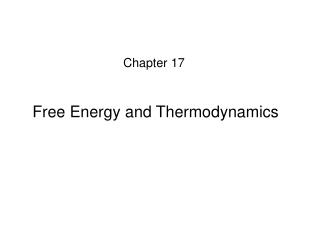DownloadDownload PresentationFree Energy and Thermodynamics

# Free Energy and Thermodynamics

Download Presentation## Free Energy and Thermodynamics

- - - - - - - - - - - - - - - - - - - - - - - - - - - E N D - - - - - - - - - - - - - - - - - - - - - - - - - - -
##### Presentation Transcript

1. Chapter 17 Free Energy and Thermodynamics

2. Spontaneity A process is said to be spontaneous if it occurs without outside intervention.

3. 800 °C 30 °C Q

4. The expansion of an ideal gas into an evacuated bulb.

5. Processes that are spontaneous in one direction are nonspontaneous in the reverse direction.

6. 800 °C 30 °C Q

7. The expansion of an ideal gas into an evacuated bulb.

8. The driving force for a spontaneous process is an increase in the entropy (S)of the universe. Entropy is a state function. It can be viewed as a measure of molecular randomness or disorder.

9. A Disordered Pile of Playing Cards

10.                               

11.                               

12. Predict the sign of ∆S • H2O(l)  H2O(g) • Ag+(aq) + Cl−(aq)  AgCl(s) • 4Fe(s) + 3O2(g)  2Fe2O3(s) • N2(g) + O2(g)  2NO(g) Try Example 17.1 on page 780

13. Entropy describes the number of microstates that are available to a system existing in a given state.

14. The expansion of an ideal gas into an evacuated bulb.

15. Possible states of four molecules in a two-bulb flask. State I State II State III Microstate: a particular arrangement of a state. State IV State V

16. Number of microstates W Probability of each state 1/16 1 State I 4 4/16 State II 6 6/16 State III most probable state 4 4/16 State IV + ) 1 1/16 State V 16

17. Statistical thermodynamics deals with the number of microstates Boltzmann’s equation: S = k lnW Boltzmann’s constant k = 1.38 x 10−23 J/K W is the number of microstates Unit of S: J/K ∆S = Sf − Si = k lnWf − k lnWi = k ln(Wf/Wi)

18. Second law of thermodynamics: In any spontaneous process there is always an increase in the entropy of the universe.

19. How to predict the spontaneity of a process? ∆Suniv > 0, spontaneous ∆Suniv < 0, nonspontaneous ∆Suniv = ∆Ssys + ∆Ssu

20. ∆Suniv = ∆Ssys + ∆Ssu

21. Reversible and Irreversible Processes In a reversible process, a system is changed in such a way that the system and the surroundings can be restored to their original states.

22. initial state final state Spontaneous processes are irreversible. Can design a reversible process that has the same initial and final states for an irreversible process.

23. For a reversible process at constant temperature units ∆S is determined only by initial and final states (because S is a state function), so we can design a reversible process to calculate ∆S.

24. Second law of thermodynamics: In any spontaneous process there is always an increase in the entropy of the universe. Another way to state the second law of thermodynamics Reversible process: ∆Suniv = 0 Irreversible process: ∆Suniv > 0

25. ∆Suniv = ∆Ssys + ∆Ssu (constant P, T)

26. Sb3S3(s) + 3Fe(s)  2Sb(s) + 3FeS(s) ∆H = −125 kJ Calculate ∆Ssu for this reaction at 25 °C and 1 atm. Try Example 17.2 on page 783

27. Possible states of four molecules in a two-bulb flask. State I State II State III Microstate: a particular arrangement of a state. State IV State V

28. Chapter 5 T↑ → S↑

29. T↑ → S↑ Where is the minimum S ?

30. Third law of thermodynamics A perfect crystal at 0 K has S = 0.

31.                               

32.                               

33. T = 0 K T > 0 K S = 0 S > 0

34. Third law of thermodynamics A perfect crystalline substance at 0 K has S = 0. Standard entropy S° : entropy of 1 mol substance at 1 atm and T (often 25 °C) Unit: J·K−1·mol−1

35. ∆Sr° = Σnp S°(product) − Σnr S°(reactant)

36. ∆Srxn° = Σnp S°(product) − Σnr S°(reactant) ∆Hrxn° = Σnp ∆Hf°(product) − Σnr ∆Hf°(reactant) The standard enthalpy of formation of a pure substance, ∆H°f, is the change in enthalpy for the reaction that forms one mole of the substance from its elements, with all substances in their standard states.

37. Calculate ∆S° for the synthesis of ammonia from N2(g) and H2(g) at 298 K: N2(g) + 3H2(g)  2NH3(g) Is this reaction spontaneous at 298 K?